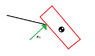# Formulation rigid body constraint

Larsen1000
Hello

I need some help with formulating the constraint force for a sliding and rotating box. The scenario is: A box is sliding down a slanted table. The center of gravity has passed the edge of the table so the box receives a counter force and torque.

I am solving the forces and moments which acts through center of gravity and therefore have formulated:

Fn = -(gravity * Rotation matrix *Constraint vector) - (Forces from external moments and rotational velocity)

The constraint vector is [0 1] which eliminates tangent forces at the edge and leaves the normal force. The point where I have problems is to formulate the constraint force caused by the rotational component. Can someone help me with this?

#### Attachments

•Constraint Force.png
1.8 KB · Views: 398